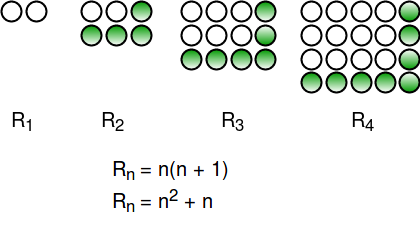# Rectangular (or Pronic) Numbers

The numbers that can be arranged to form a rectangle are called Rectangular Numbers (also known as Pronic numbers). The first few rectangular numbers are:
0, 2, 6, 12, 20, 30, 42, 56, 72, 90, 110, 132, 156, 182, 210, 240, 272, 306, 342, 380, 420, 462 . . . . . .

Given a number n, find n-th rectangular number.

Examples:

```Input : 1
Output : 2

Input : 4
Output : 20

Input : 5
Output : 30
```

## Recommended: Please try your approach on {IDE} first, before moving on to the solution.

The number 2 is a rectangular number because it is 1 row by 2 columns. The number 6 is a rectangular number because it is 2 rows by 3 columns, and the number 12 is a rectangular number because it is 3 rows by 4 columns.

If we observe these numbers carefully, we can notice that n-th rectangular number is n(n+1).## C++

 `// CPP Program to find n-th rectangular number ` `#include ` `using` `namespace` `std; ` ` `  `// Returns n-th rectangular number ` `int` `findRectNum(``int` `n) ` `{ ` `    ``return` `n * (n + 1); ` `} ` ` `  `// Driver code ` `int` `main() ` `{ ` `    ``int` `n = 6; ` `    ``cout << findRectNum(n); ` `    ``return` `0; ` `} `

## Java

 `// Java Program to find n-th rectangular number ` `import` `java.io.*; ` ` `  `class` `GFG { ` ` `  `    ``// Returns n-th rectangular number ` `    ``static` `int` `findRectNum(``int` `n) ` `    ``{ ` `        ``return` `n * (n + ``1``); ` `    ``} ` ` `  `    ``// Driver code ` `    ``public` `static` `void` `main(String[] args) ` `    ``{ ` `        ``int` `n = ``6``; ` `        ``System.out.println(findRectNum(n)); ` `    ``} ` `} ` ` `  `// This code is contributed by vt_m. `

## C#

 `// C# Program to find n-th rectangular number ` ` `  `using` `System; ` ` `  `class` `GFG { ` ` `  `    ``// Returns n-th rectangular number ` `    ``static` `int` `findRectNum(``int` `n) ` `    ``{ ` `        ``return` `n * (n + 1); ` `    ``} ` ` `  `    ``// Driver code ` `    ``public` `static` `void` `Main() ` `    ``{ ` `        ``int` `n = 6; ` `        ``Console.Write(findRectNum(n)); ` `    ``} ` `} ` ` `  `// This code is contributed by vt_m. `

## Python

 `# Python3 Program to find n-th rectangular number ` ` `  `# Returns n-th rectangular number ` `def` `findRectNum(n): ` `    ``return` `n``*``(n ``+` `1``) ` ` `  `# Driver code  ` `n ``=` `6` `print` `(findRectNum(n)) ` ` `  `# This code is contributed by Shreyanshi Arun. `

## PHP

 ` `

Output:

```42
```

Check if a given number is Pronic | Efficient Approach

This article is contributed by DANISH_RAZA . If you like GeeksforGeeks and would like to contribute, you can also write an article using contribute.geeksforgeeks.org or mail your article to contribute@geeksforgeeks.org. See your article appearing on the GeeksforGeeks main page and help other Geeks.

GeeksforGeeks has prepared a complete interview preparation course with premium videos, theory, practice problems, TA support and many more features. Please refer Placement 100 for details

My Personal Notes arrow_drop_up

Improved By : jit_t

Article Tags :
Practice Tags :

Be the First to upvote.

Please write to us at contribute@geeksforgeeks.org to report any issue with the above content.